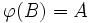# Local finiteness is quotient-closed

This article gives the statement, and possibly proof, of a group property (i.e., locally finite group) satisfying a group metaproperty (i.e., quotient-closed group property)
View all group metaproperty satisfactions | View all group metaproperty dissatisfactions |Get help on looking up metaproperty (dis)satisfactions for group properties
Get more facts about locally finite group |Get facts that use property satisfaction of locally finite group | Get facts that use property satisfaction of locally finite group|Get more facts about quotient-closed group property

## Statement

Any quotient group of a locally finite group is also locally finite. In other words, if$\varphi:G \to H$ is a surjective homomorphism, and$G$ is locally finite, so is$H$.

## Definitions used

### Locally finite group

Further information: Locally finite group

A group is termed locally finite if every finitely generated subgroup of it is finite.

## Proof

Given: A locally finite group$G$, a surjective homomorphism$\varphi:G \to H$.

To prove: If$A$ is a finite subset of$H$,$\langle A \rangle$ is finite.

Proof:

1. Construction of a finite set$B \subseteq G$ such that$\varphi(B) = A$: Since$\varphi$ is surjective, we can pick, for each$a \in A$, an element$b \in G$ such that$\varphi(b) = a$. Making such a choice for each$a \in A$, we get a finite subset$B$ of$G$ such that$\varphi(B) = A$.
2.$\varphi(\langle B \rangle) = \langle \varphi(B) \rangle = \langle A \rangle$: This follows from the fact that$\varphi$ is a homomorphism.
3.$\langle B \rangle$ is a finite group (Given data used:$G$ is locally finite): Since$G$ is locally finite, and$B$ is a finite subset,$\langle B \rangle$ is a finite group.
4.$\langle A \rangle$ is finite: By steps (3) and (4),$\langle A \rangle$ is the image of a finite group, and hence, is finite.